Project in progress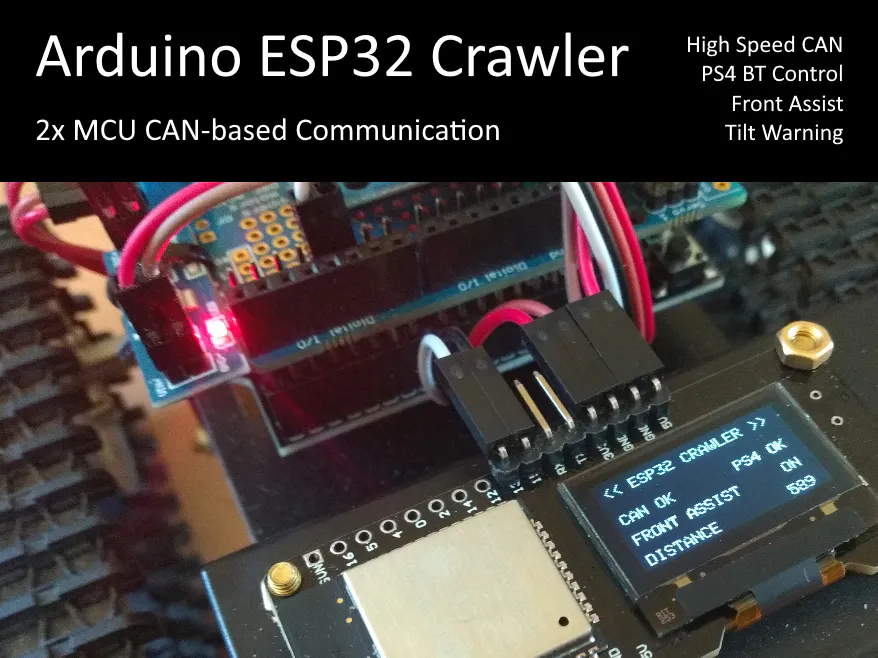A simple Arduino/ESP32 crawler with PS4 BT remote control, but with 2x MCU and an advanced CAN-based communication ("drive-by-wire").

• 7,222 views
• 3 respects

## Components and supplies

 DOIT T101 Mini
×1
 Wemos D1 R32 TT-GO variant uses Micro-USB instead of type A on Wemos variant.
×1
×1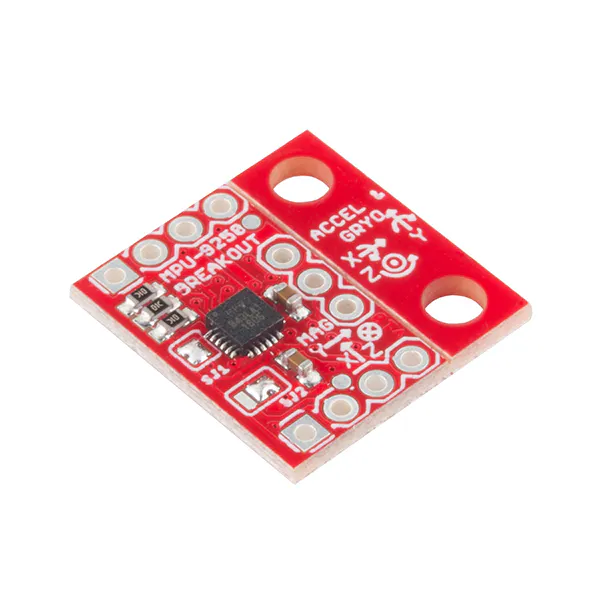SparkFun IMU Breakout - MPU-9250 9-DOF IMU
×1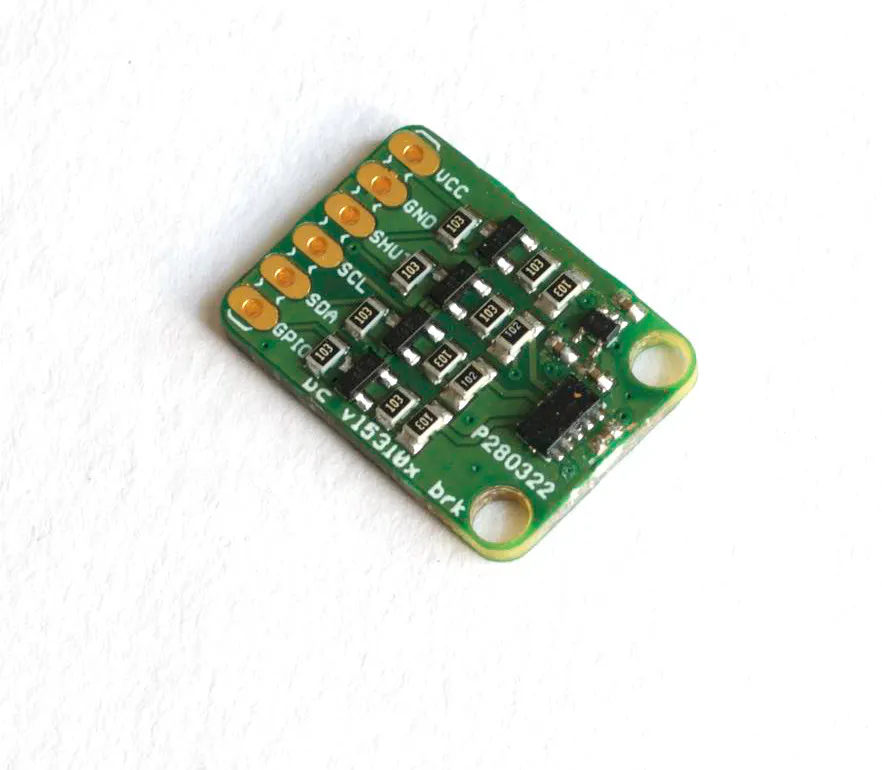ProtoCentral Electronics ProtoCentral VL53L0X Laser ToF Sensor breakout board
×1
 D-duino32
×1
 AMS1117 5.0V
×1
 SN65HVD230 HS CAN Transceiver
×2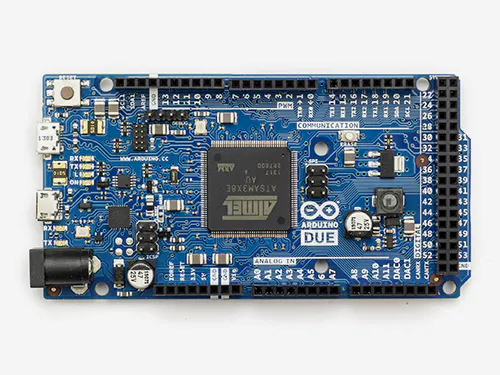Arduino Due Used as CAN monitor
×1
 SN65HVD230 Connected to Arduino Due
×1Jumper wires (generic)
×1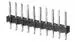Male Header 40 Position 1 Row (0.1")
×1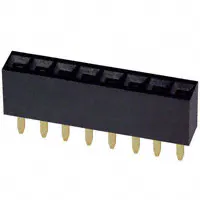Female Header 8 Position 1 Row (0.1")
×5

## Apps and online services

An Arduino based crawler/tank!? Haven't we already seen dozens of them? Maybe, but not such a variant. The focus is lesser about the crawler and more about communication between electronic control units (ECUs) based on Controller Area Network (CAN) as used in current automobiles.

• Implementation updated for both boards
• A couple of fixes inlcuded to increase stability for I2C and TOF (took quite a while)
• Reduced the CPU frequency of the D-duino32 from 240 to 160 MHz (reduced heat emission and more important power consumtion in order to lesser stress the supply)
• Learning A: Don't try to read more than every 50ms the TOF sensor (fixed freezes)
• Learning B: Don't read to quickly from I2C devices (fixed instabilities by 10ms delay)
• ESP32-CAM with folding device included (it's more a gimick rather a feature, it only shares the 5V power supply from DC regulator, but it's not connected to CAN)
• Learning C: If the video of the ESP32-CAM is disturbed, check if sufficient power is supplied (on-board 5V from Wemos D1 R32 was insufficient)

Fore sure: First off, we need a vehicle - messing around on CAN in a real car isn't a good idea - and some spare parts from my loot boxes. I picked up a tracked chassis with 2 motors from DOIT (T101 mini), a Wemos D1 R32, a D-duino32 with on-board OLED, a motor shield from Adafruit, a TOF sensor (VL53L0X) for distance measurements, an IMU (MPU9250) for tilt detection, a battery box, some screws & nuts, jump wires and last but not least two CAN transceivers (SN65HVD230). After a small while everything was assembled. So let's have a look what came out of the spare time activity...

But what is now about the CAN communication? Before we have a closer look at it, I would like give an overview about the wiring used for this build. Here the major focus is the high-speed (HS) CAN connection - whereby "high-speed" tells us more about the electrical behaviour rather than the baudrate. The ESP32 has got an integrated HS CAN controller, so we just need to hook up a matching CAN transceiver on both MCUs, compare here.

The used SN65HVD230 transceivers need to be powered by 3.3V and are using the same as signal level. They are going to be connected from their CRX and CTX to GPIOs of the MCUs (no cross-over). Afterwards both are connected to each other via CANH and CANL (no cross-over). Motor shield, TOF/IMU sensor are connected to the D1 R32 by I2C (SDA & SCL). The battery box and motors are connected to the shield and finally the D-duino32 is power by the help of a DC regulator (AMS1117-5.0V). I tried it by using V5 & GND from D1 R32, but struggled a lot with strange behaviours (especially about the CAN communication). After applying battery power by the help of the regulator directly to the D-duni32, the problems were gone. I guess the voltage regulator of the D1 R32 is just not capable enough to drive the shield, sensors plus the D-duino32.

The comminication over CAN between the ECUs happens by frames. Here I used the standard format which uses up to 8 bytes per message as payload. If you are interrested how the bus abritration per ECU is handled and how the complete frame formate looks like, please have a look here. The messages (or frames) are differentiated by network-wide unqiue IDs. This ID is also equivilant with a priority (smaller ID has higher priority in case of concurrent abritration). So already by creating a message catalog some care is needed also for the order of IDs and not only payload.

In the project IDs 0x10, 0x11 and 0x12 are used to transfer the information from the PS4 controller connected to D-duino32 over CAN to D1 R32 which calculates the steering/throttle and finally the left and right motor speed from it. Vise versa D1 R32 sends a messages with ID 0x20 containing a status of the front assistant system (autonomious breaking based on distance in front collected by the TOF sensor), status of tilt warning system (pitch and/or roll > 45° gathered by IMU). Status information is shown on the OLED and used to control the PS4 LED and rumble function for a direct feedback to the "driver".

``````void task_txCAN(void * pvParameters) {
for(;;) {
CAN_frame_t tx_frame;
int d = distance;
tx_frame.FIR.B.FF==CAN_frame_std;
tx_frame.MsgID = 0x20;
tx_frame.FIR.B.DLC = 8;
tx_frame.data.u8 = byte(auto_break);
tx_frame.data.u8 = byte(break_active);
tx_frame.data.u8 = byte(tilt_warn);
tx_frame.data.u8 = 0;
tx_frame.data.u8 = byte(d>>(3*8));
tx_frame.data.u8 = byte(d<<(1*8)>>(3*8));
tx_frame.data.u8 = byte(d<<(2*8)>>(3*8));
tx_frame.data.u8 = byte(d<<(3*8)>>(3*8));
ESP32Can.CANWriteFrame(&tx_frame);
delay(50);
}
}
``````

Often an information needs to be converted in order to be transfered by bytes in a frame. This could happen by converting e.g. a bool to a bit (if there is no shortage, you can also use a byte). More care is need for other data types and even more how those data types are represented on a specific MCU. As example: An Arduino Uno is using 2 bytes for an integer (16-bit), but the ESP32 is using 4 bytes (32-bit). Special care is needed if different MCU architectures are mixed in a network and how the data type representation for each of them is looking like. Therefore it's important to know how the message catalog looks like and how presenatation rules for the payload need to be handled.

For analyzing/monitoring the traffic between D1 R32 and D-Duino32, I used an Arduino Due which also owns an integrated CAN controller. The Due - different from EPS32 - supports not only one but two channels, so you can connect two transceivers and e.g. listen to two different CAN network segments. Quite handy if a gateway needs to be analyzed, but not needed in this project. Here is one simple segment between only two MCUs all we need. The transceiver of the Due can simply be hooked up on CANH and CANL to the existing wiring, because CAN is a bus and not a point-to-point connection.

The Due receives all messages on the bus (there is no filter applied to it) and acts as read-only spy. The received frames are printed to USB serial and on a PC PuTTY is used to log them.

Long story short: I hope you had some fun while reading through this article. But now let's have a ride with the crawler in the living room... CAN I do?!

Here are some more impressions with the camera add-on board. The camera is using the example implemenation from the Arduino ESP32 SDK. Only change to it was to use WiFi AP instead of STA. Now the camera opens an own access point where a mobile phone can connect to. On my Android phone, I used Chrome to show the HTML page with embedded video stream.

## Code

##### Wemos-D1-R32_Tank_T101_CAN_VL53L0X_MPU9250.inoArduino
```// << ESP32 Crawler >>
// board is "ESP32 Dev Module"
// CAN Driver https://github.com/nhatuan84/esp32-can-protocol-demo
// VL53L0X Driver from Pololu via Library Manager
// MPU9250 Driver from Bolder Flight System via Library Manager
// Adafruit Motor Shield V2 via Library Manager

#define LED_PIN 2

#include <Wire.h>

#define SPEED_MIN 70 // motor min
#define SPEED_MAX 255 // max speed
#define SPEED_NONE 0
int constSpeeds[] = {100, 150, 200, 250};
int speedIndex = 1;
bool incState = false;
bool incLast = false;
bool decState = false;
bool decLast = false;

#include <VL53L0X.h>
VL53L0X TOF;
#define TOF_INTERVALL 50 // ms
unsigned long lastTOFmeasurement = 0;

#define DISTANCE_MIN 170
int distance = 0;
bool auto_break  = true;
bool break_active = false;

#include "MPU9250.h"
MPU9250 IMU(Wire, 0x68);
#define TILT_MAX 45
bool tilt_warn = false;

#include <ESP32CAN.h>
#define CAN_SPEED CAN_SPEED_250KBPS
#define CAN_TX GPIO_NUM_16
#define CAN_RX GPIO_NUM_17
#include <CAN_config.h>

CAN_device_t CAN_cfg;
const int rx_queue_size = 10;

#define STICK_CAN_TIMEOUT 250
unsigned long lastStickReveiced;

int stick_left_x = 0;
int stick_left_y = 0;
int stick_right_x = 0;
int stick_right_y = 0;

bool button_left = false;
bool button_right = false;
bool button_up = false;
bool button_down = false;

bool button_square = false;
bool button_circle = false;
bool button_triangle = false;
bool button_cross = false;

bool button_l1 = false;
bool button_r1 = false;

for(;;) {
CAN_frame_t rx_frame;

if(rx_frame.FIR.B.FF==CAN_frame_std) { // standard frame format
digitalWrite(LED_PIN, HIGH);
// start of message evaluation
if (rx_frame.MsgID == 0x10 && rx_frame.FIR.B.DLC == 8) {
if (rx_frame.data.u8) {
stick_right_x = int(rx_frame.data.u8);
}
else {
stick_right_x = int(-(rx_frame.data.u8 + 1));
}
if (rx_frame.data.u8) {
stick_right_y = int(rx_frame.data.u8);
}
else {
stick_right_y = int(-(rx_frame.data.u8 + 1));
}
if (rx_frame.data.u8) {
stick_left_x = int(rx_frame.data.u8);
}
else {
stick_left_x = int(-(rx_frame.data.u8 + 1));
}
if (rx_frame.data.u8) {
stick_left_y = int(rx_frame.data.u8);
}
else {
stick_left_y = int(-(rx_frame.data.u8 + 1));
}
lastStickReveiced = millis();
}
else if (rx_frame.MsgID == 0x11 && rx_frame.FIR.B.DLC == 8) {
button_right = (bool)rx_frame.data.u8;
button_left = (bool)rx_frame.data.u8;
button_up = (bool)rx_frame.data.u8;
button_down = (bool)rx_frame.data.u8;
button_square = (bool)rx_frame.data.u8;
button_circle = (bool)rx_frame.data.u8;
button_triangle = (bool)rx_frame.data.u8;
button_cross = (bool)rx_frame.data.u8;
}
else if (rx_frame.MsgID == 0x12 && rx_frame.FIR.B.DLC == 8) {
button_r1 = (bool)rx_frame.data.u8;
button_l1 = (bool)rx_frame.data.u8;
}
// end of message evaluation
}
}
else {
digitalWrite(LED_PIN, LOW);
}
}
}

for(;;) {
CAN_frame_t tx_frame;
int d = distance;

tx_frame.FIR.B.FF==CAN_frame_std;
tx_frame.MsgID = 0x20;
tx_frame.FIR.B.DLC = 8;
tx_frame.data.u8 = byte(auto_break);
tx_frame.data.u8 = byte(break_active);
tx_frame.data.u8 = byte(tilt_warn);
tx_frame.data.u8 = 0;
tx_frame.data.u8 = byte(d>>(3*8));
tx_frame.data.u8 = byte(d<<(1*8)>>(3*8));
tx_frame.data.u8 = byte(d<<(2*8)>>(3*8));
tx_frame.data.u8 = byte(d<<(3*8)>>(3*8));

ESP32Can.CANWriteFrame(&tx_frame);

delay(50);
}
}

void setup()
{
//  Serial.begin(57600);

pinMode(LED_PIN, OUTPUT);
digitalWrite(LED_PIN, HIGH);

Wire.begin();

AFMS.begin();

right_motor->setSpeed(SPEED_NONE);
right_motor->run(RELEASE);

left_motor->setSpeed(SPEED_NONE);
left_motor->run(RELEASE);

TOF.setTimeout(500);
if (!TOF.init()) {
//    Serial.println("TOF init failed!");
while (1) {
digitalWrite(LED_PIN, LOW);
delay(500);
digitalWrite(LED_PIN, HIGH);
delay(500);
}
}
TOF.startContinuous();

if (IMU.begin() < 0) {
//    Serial.println("IMU init failed!");
while (1) {
digitalWrite(LED_PIN, LOW);
delay(1000);
digitalWrite(LED_PIN, HIGH);
delay(1000);
}
}

CAN_cfg.speed=CAN_SPEED;
CAN_cfg.tx_pin_id = CAN_TX;
CAN_cfg.rx_pin_id = CAN_RX;
CAN_cfg.rx_queue = xQueueCreate(rx_queue_size, sizeof(CAN_frame_t));

ESP32Can.CANInit();

digitalWrite(LED_PIN, LOW);

4096,      /* Stack size in words */
NULL,      /* Task input parameter */
1,         /* Priority of the task */
1);        /* Core where the task should run */

4096,      /* Stack size in words */
NULL,      /* Task input parameter */
1,         /* Priority of the task */
1);        /* Core where the task should run */

lastStickReveiced = millis();
}

void loop()
{
//  Serial.print("R_X: "); Serial.print(stick_right_x, DEC);
//  Serial.print(" R_Y: "); Serial.print(stick_right_y, DEC);
//  Serial.print(" L_X: "); Serial.print(stick_left_x, DEC);
//  Serial.print(" L_Y: "); Serial.print(stick_left_y, DEC);
//  Serial.println();

float acX, acY, acZ;
float p, r;
int pitch = 0, roll = 0;

acX = IMU.getAccelX_mss();
acY = IMU.getAccelY_mss();
acZ = IMU.getAccelZ_mss();
p = atan(acX / sqrt((acY * acY) + (acZ * acZ)));
r = atan(acY / sqrt((acX * acX) + (acZ * acZ)));
pitch = int(p * 57.2958); // 180.0 / pi
roll = int(r * 57.2958); // 180.0 / pi
tilt_warn = max(abs(pitch), abs(roll)) > TILT_MAX;
//  Serial.print("IMUP: "); Serial.print(pitch);
//  Serial.print(" IMUR: "); Serial.print(roll);
//  Serial.println();

delay(10);

// read TOF only once per intervall
if (millis() - lastTOFmeasurement >= TOF_INTERVALL) {
if (TOF.timeoutOccurred() || distance > 8191) distance = 8190;
lastTOFmeasurement = millis();
delay(10);
}
//  Serial.print("DIS: "); Serial.print(distance); Serial.print(" ");
//  Serial.println();

if ((millis() - lastStickReveiced) < STICK_CAN_TIMEOUT) {

int throttle = stick_left_y; // -128 0 127
int steering = stick_right_x; // -128 0 127
int left_speed = 0, right_speed = 0;

if (throttle > 0) throttle +=1; // max out to 128
if (steering > 0) steering +=1; // max out to 128

if (button_triangle) {
auto_break = true;
}
else if (button_cross) {
auto_break = false;
}

// mix throttle and steering inputs to obtain left & right motor speeds
left_speed = ((throttle * SPEED_MAX / 128) + (steering * SPEED_MAX / 128));
right_speed = ((throttle * SPEED_MAX / 128) - (steering * SPEED_MAX / 128));

// cap speeds to max
left_speed = min(max(left_speed, -SPEED_MAX), SPEED_MAX);
right_speed = min(max(right_speed, -SPEED_MAX), SPEED_MAX);

// decrease/increase fixed speed for digital pad control)
decState = button_l1;
if(decState && decState != decLast) {
if (speedIndex > 0) speedIndex--;
}
decLast = decState;
incState = button_r1;
if(incState && incState != incLast) {
if (speedIndex < 3) speedIndex++;
}
incLast = incState;

if (button_right){
left_speed = constSpeeds[speedIndex];
right_speed = -constSpeeds[speedIndex];
}
else if (button_left){
left_speed = -constSpeeds[speedIndex];
right_speed = constSpeeds[speedIndex];
}
else if (button_up) {
left_speed = constSpeeds[speedIndex];
right_speed = constSpeeds[speedIndex];
}
else if (button_down){
left_speed = -constSpeeds[speedIndex];
right_speed = -constSpeeds[speedIndex];
}

if (abs(left_speed) < SPEED_MIN) left_speed = 0;
if (abs(right_speed) < SPEED_MIN) right_speed = 0;

if (auto_break) {
break_active = false;
if (distance < (DISTANCE_MIN * 1.5) && left_speed > (SPEED_MAX / 2) && right_speed > (SPEED_MAX / 2)) {
left_speed = left_speed / 2;
right_speed = right_speed / 2;
}
if (distance < DISTANCE_MIN && left_speed > 0 && right_speed > 0) {
left_speed = SPEED_NONE;
right_speed = SPEED_NONE;
break_active = true;
}
}

//    Serial.print("RSDP: "); Serial.print(right_speed);
//    Serial.print(" LSPD: "); Serial.print(left_speed);
//    Serial.println();

right_motor->setSpeed(abs(right_speed));
if (right_speed >= 0) right_motor->run(FORWARD);
else right_motor->run(BACKWARD);

left_motor->setSpeed(abs(left_speed));
if (left_speed >= 0) left_motor->run(FORWARD);
else left_motor->run(BACKWARD);

delay(10);
}
else {
//    Serial.println("TIMEOUT: Stick Frame not received");

stick_left_x = 0;
stick_left_y = 0;
stick_right_x = 0;
stick_right_y = 0;

button_left = false;
button_right = false;
button_up = false;
button_down = false;

button_square = false;
button_circle = false;
button_triangle = false;
button_cross = false;

button_l1 = false;
button_r1 = false;

right_motor->setSpeed(SPEED_NONE);
right_motor->run(RELEASE);

left_motor->setSpeed(SPEED_NONE);
left_motor->run(RELEASE);

delay(STICK_CAN_TIMEOUT/2);
}
}
```
##### D-duino-32_Tank_T101_CAN_PS4_OLED.inoArduino
```// << ESP32 Crawler >>
// board is "D-duino-32"
// CAN Driver https://github.com/nhatuan84/esp32-can-protocol-demo
// PS4 Driver https://github.com/NURobotics/PS4-esp32
// PS4 MAC https://dancingpixelstudios.com/sixaxis-controller/sixaxispairtool/
// in case the controller is no longer pairing but was working before,
// run "esptool --chip esp32 erase_flash" in C:\Users\<user>\AppData\Local\Arduino15\packages\esp32\tools\esptool_py\2.6.1
// see https://github.com/NURobotics/PS4-esp32/issues/2#issuecomment-602852034

#include <Wire.h>
#include <Adafruit_SSD1306.h> // works only if board and variant config for D-duino-32 is available

#define SCREEN_WIDTH 128 // OLED display width, in pixels
#define SCREEN_HEIGHT 64 // OLED display height, in pixels

#define OLED_RESET 3
#define OLED_ROTATION 0

#if (SSD1306_LCDHEIGHT != 64)
#endif

#include <PS4Controller.h>
#define PS4_HOST_MAC "00:1e:ab:4c:4e:c8"
#define TILT_RUMBLE 127

#include <ESP32CAN.h>
#define CAN_SPEED CAN_SPEED_250KBPS
#define CAN_TX GPIO_NUM_15
#define CAN_RX GPIO_NUM_13
#include <CAN_config.h>

CAN_device_t CAN_cfg;
const int rx_queue_size = 10;

#define STATUS_CAN_TIMEOUT 250
unsigned long lastStatusReveiced;

bool auto_break = false;
bool break_active = false;
int distance = 0;
byte tilt_warn = false;

for(;;) {
CAN_frame_t rx_frame;

// start of message evaluation
if(rx_frame.FIR.B.FF==CAN_frame_std) { // standard frame format
if (rx_frame.MsgID == 0x20 && rx_frame.FIR.B.DLC == 8) {
auto_break =   bool(rx_frame.data.u8);
break_active = bool(rx_frame.data.u8);
tilt_warn =    bool(rx_frame.data.u8);
distance  =     int(rx_frame.data.u8)<<(3*8);
distance +=     int(rx_frame.data.u8)<<(2*8);
distance +=     int(rx_frame.data.u8)<<(1*8);
distance +=     int(rx_frame.data.u8);

lastStatusReveiced = millis();
}
}
// end of message evaluation
}
}
}

void setup()
{
setCpuFrequencyMhz(160); // reduce heat emission & power consumption (default would be 240Mhz)

//  Serial.begin(57600);

if(!display.begin(SSD1306_SWITCHCAPVCC, 0x3c)) { // 0x3c for on-board OLED of D-duino-32
//    Serial.println(F("SSD1306 allocation failed"));
while (1) {}
}
display.clearDisplay();
display.setTextSize(1);
display.setTextColor(WHITE, BLACK);
display.setCursor(5,5);
display.print("<< ESP32 CRAWLER >>");
display.display();

PS4.begin(PS4_HOST_MAC);

CAN_cfg.speed=CAN_SPEED;
CAN_cfg.tx_pin_id = CAN_TX;
CAN_cfg.rx_pin_id = CAN_RX;
CAN_cfg.rx_queue = xQueueCreate(rx_queue_size, sizeof(CAN_frame_t));

ESP32Can.CANInit();

4096,      /* Stack size in words */
NULL,      /* Task input parameter */
1,         /* Priority of the task */
1);        /* Core where the task should run */

lastStatusReveiced = 0;
}

void loop()
{
if(PS4.isConnected()) {

//    Serial.print("R_X: "); Serial.print(PS4.data.analog.stick.rx, DEC);
//    Serial.print(" R_Y: "); Serial.print(PS4.data.analog.stick.ry, DEC);
//    Serial.print(" L_X: "); Serial.print(PS4.data.analog.stick.lx, DEC);
//    Serial.print(" L_Y: "); Serial.print(PS4.data.analog.stick.ly, DEC);
//    Serial.println();

//    Serial.print("DIS: "); Serial.println(distance);

display.setCursor(83,25);
display.print("PS4 OK ");

display.setCursor(5, 40);
if (auto_break) {
if (break_active) {
display.print("FRONT ASSIST ACTIVE");
PS4.setLed(255, 0, 0);
}
else {
display.print("FRONT ASSIST     ON ");
PS4.setLed(0, 255, 0);
}
}
else {
display.print("FRONT ASSIST     OFF");
PS4.setLed(0, 0, 255);
}

#ifdef TILT_RUMBLE
if (tilt_warn) {
PS4.setRumble(0, TILT_RUMBLE);
}
else {
PS4.setRumble(0, 0);
}
#endif

// PS4.sendToController(); // moved after sending frames
// delay(15); // replaced by delay between frames

display.setCursor(5, 55);
display.print("DISTANCE");
char sf;
sprintf(sf, "%4d", distance);
display.setCursor(95, 55);
display.print(sf);

int rgt_stick_x = PS4.data.analog.stick.rx; // -128 0 127
int rgt_stick_y = PS4.data.analog.stick.ry; // -128 0 127
int lft_stick_x = PS4.data.analog.stick.lx; // -128 0 127
int lft_stick_y = PS4.data.analog.stick.ly; // -128 0 127

// prepare analog sticks (bytes)
byte b_rgt_stick_x_val;
byte b_rgt_stick_x_dir;
if (rgt_stick_x >= 0) {
b_rgt_stick_x_val = byte(rgt_stick_x);
b_rgt_stick_x_dir = 1;
}
else {
b_rgt_stick_x_val = byte(abs(rgt_stick_x)-1);
b_rgt_stick_x_dir = 0;
}

byte b_rgt_stick_y_val;
byte b_rgt_stick_y_dir;
if (rgt_stick_y >= 0) {
b_rgt_stick_y_val = byte(rgt_stick_y);
b_rgt_stick_y_dir = 1;
}
else {
b_rgt_stick_y_val = byte(abs(rgt_stick_y)-1);
b_rgt_stick_y_dir = 0;
}

byte b_lft_stick_x_val;
byte b_lft_stick_x_dir;
if (lft_stick_x >= 0) {
b_lft_stick_x_val = byte(lft_stick_x);
b_lft_stick_x_dir = 1;
}
else {
b_lft_stick_x_val = byte(abs(lft_stick_x)-1);
b_lft_stick_x_dir = 0;
}

byte b_lft_stick_y_val;
byte b_lft_stick_y_dir;
if (lft_stick_y >= 0) {
b_lft_stick_y_val = byte(lft_stick_y);
b_lft_stick_y_dir = 1;
}
else {
b_lft_stick_y_val = byte(abs(lft_stick_y)-1);
b_lft_stick_y_dir = 0;
}

CAN_frame_t tx_control;

// Send analog sticks bytes on CAN
tx_control.FIR.B.FF==CAN_frame_std;
tx_control.MsgID = 0x10;
tx_control.FIR.B.DLC = 8;
tx_control.data.u8 = b_rgt_stick_x_val;
tx_control.data.u8 = b_rgt_stick_x_dir;
tx_control.data.u8 = b_rgt_stick_y_val;
tx_control.data.u8 = b_rgt_stick_y_dir;
tx_control.data.u8 = b_lft_stick_x_val;
tx_control.data.u8 = b_lft_stick_x_dir;
tx_control.data.u8 = b_lft_stick_y_val;
tx_control.data.u8 = b_lft_stick_y_dir;

ESP32Can.CANWriteFrame(&tx_control);

delay(5);

// send button states as byte on CAN
tx_control.FIR.B.FF==CAN_frame_std;
tx_control.MsgID = 0x11;
tx_control.FIR.B.DLC = 8;
tx_control.data.u8 = byte(PS4.data.button.right);
tx_control.data.u8 = byte(PS4.data.button.left);
tx_control.data.u8 = byte(PS4.data.button.up);
tx_control.data.u8 = byte(PS4.data.button.down);
tx_control.data.u8 = byte(PS4.data.button.square);
tx_control.data.u8 = byte(PS4.data.button.circle);
tx_control.data.u8 = byte(PS4.data.button.triangle);
tx_control.data.u8 = byte(PS4.data.button.cross);
ESP32Can.CANWriteFrame(&tx_control);

delay(5);

// send button states as byte on CAN
tx_control.FIR.B.FF==CAN_frame_std;
tx_control.MsgID = 0x12;
tx_control.FIR.B.DLC = 8;
tx_control.data.u8 = byte(PS4.data.button.r1);
tx_control.data.u8 = byte(PS4.data.button.l1);
tx_control.data.u8 = byte(PS4.data.button.r2);
tx_control.data.u8 = byte(PS4.data.analog.button.r2);
tx_control.data.u8 = byte(PS4.data.button.l2);
tx_control.data.u8 = byte(PS4.data.analog.button.l2);
tx_control.data.u8 = byte(PS4.data.button.r3);
tx_control.data.u8 = byte(PS4.data.button.l3);
ESP32Can.CANWriteFrame(&tx_control);

delay(5);

PS4.sendToController(); // should not be done more than each 15ms, but with delay (5+5+5) from tx CAN should be ok
}
else {
//    Serial.println("No PS4 controller connected.");
display.setCursor(83,25);
display.print("PS4 NOK");
display.setCursor(5, 40);
display.print("                    ");
display.setCursor(5, 55);
display.print("                    ");
display.display();

// delay(5);

CAN_frame_t tx_control;

// Send analog sticks bytes on CAN
tx_control.FIR.B.FF==CAN_frame_std;
tx_control.MsgID = 0x10;
tx_control.FIR.B.DLC = 8;
tx_control.data.u8 = 0;
tx_control.data.u8 = 0;
tx_control.data.u8 = 0;
tx_control.data.u8 = 0;
tx_control.data.u8 = 0;
tx_control.data.u8 = 0;
tx_control.data.u8 = 0;
tx_control.data.u8 = 0;
ESP32Can.CANWriteFrame(&tx_control);

delay(5);

// send button states as byte on CAN
tx_control.FIR.B.FF==CAN_frame_std;
tx_control.MsgID = 0x11;
tx_control.FIR.B.DLC = 8;
tx_control.data.u8 = 0;
tx_control.data.u8 = 0;
tx_control.data.u8 = 0;
tx_control.data.u8 = 0;
tx_control.data.u8 = 0;
tx_control.data.u8 = 0;
tx_control.data.u8 = 0;
tx_control.data.u8 = 0;
ESP32Can.CANWriteFrame(&tx_control);

delay(5);

// send button states as byte on CAN
tx_control.FIR.B.FF==CAN_frame_std;
tx_control.MsgID = 0x12;
tx_control.FIR.B.DLC = 8;
tx_control.data.u8 = 0;
tx_control.data.u8 = 0;
tx_control.data.u8 = 0;
tx_control.data.u8 = 0;
tx_control.data.u8 = 0;
tx_control.data.u8 = 0;
tx_control.data.u8 = 0;
tx_control.data.u8 = 0;
ESP32Can.CANWriteFrame(&tx_control);

delay(5);
}
if ((millis() - lastStatusReveiced) < STATUS_CAN_TIMEOUT) {
display.setCursor(5,25);
display.print("CAN OK ");
}
else {
auto_break = false;
break_active = false;
distance = 0;
tilt_warn = false;
display.setCursor(5,25);
display.print("CAN NOK");
display.setCursor(5, 40);
display.print("                    ");
display.setCursor(5, 55);
display.print("                    ");
delay(15);
}
display.display();
}
```

#### Published on

April 22, 2020
See similar projects
you might like

#### Arduino Powered CPR Feedback Device

Project showcase by David Escobar

• 10,252 views
• 36 respects

Project tutorial by Kutluhan Aktar

• 7,814 views
• 17 respects

#### Solar Powered LED Parking Sensor

Project tutorial by aams86

• 5,085 views
• 15 respects

Project tutorial by Manivannan

• 11,783 views
• 64 respects

#### Raspberry Pi - Powered Candy Dispenser

Project tutorial by Arduino “having11” Guy

• 10,914 views
• 1 comment
• 16 respects

#### UV-C Based Germs Disinfecting Machine

Project tutorial by Yeshvanth Muniraj

• 6,685 views